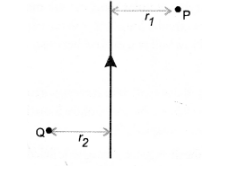# AB is a current carrying conductor

Question:

AB is a current carrying conductor in the plane of the paper as shown in figure. What are the directions of magnetic fields produced by it at points P and

Q ? Given, r1 > r2, where will the strength of the magnetic field be larger ?

(CBSE 2010, 2012)Solution:

According to Right hand thumb rule, the direction of the mag¬netic field is into the plane of the paper at P and the direction of the magnetic field is

out of the plane of the paper at Q.

The strength of the magnetic field due to a current carrying conductor decreases with increase in distance (i.e. B ∝ 1/r ).

Therefore, strength of the magnetic field will be larger at point Q than at point P.